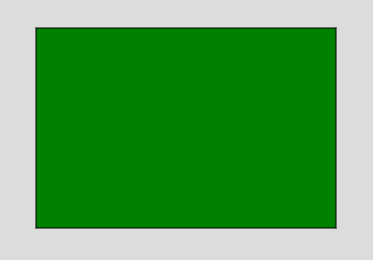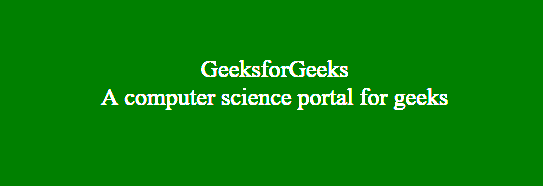# p5.js | draw() Function

• Last Updated : 23 Aug, 2019

The draw() function is called after setup() function. The draw() function is used to executes the code inside the block until the program is stopped or noLoop() is called. If the program does not contain noLoop() function within setup() function then draw() function will still be executed once before stopping it. It should always be controlled with noLoop(), redraw() and loop() functions.

Syntax:

Hey geek! The constant emerging technologies in the world of web development always keeps the excitement for this subject through the roof. But before you tackle the big projects, we suggest you start by learning the basics. Kickstart your web development journey by learning JS concepts with our JavaScript Course. Now at it's lowest price ever!

`draw()`

Below examples illustrate the draw() function in p5.js:

Example 1:

 `function` `setup() { ``     ` `    ``// Create Canvas of given size ``    ``createCanvas(400, 300); ``} `` ` `function` `draw() { ``     ` `    ``background(220); ``     ` `    ``// Use color() function ``    ``let c = color(``'green'``); `` ` `    ``// Use fill() function to fill color ``    ``fill(c); ``     ` `    ``// Draw a rectangle ``    ``rect(50, 50, 300, 200); ``     ` `} `

Output:Example 2:

 `function` `setup() {  ``    ` `    ``// Create Canvas of given size ``    ``var` `cvs = createCanvas(600, 250);``}`` ` `function` `draw() {``   ` `    ``// Set the background color``    ``background(``'green'``); ``   ` `    ``// Use createDiv() function to``    ``// create a div element``    ``var` `myDiv = createDiv(``'GeeksforGeeks'``);``   ` `    ``var` `myDiv1 = createDiv(``'A computer science portal for geeks'``);``   ` `    ``// Use child() function``    ``myDiv.child(myDiv1);``   ` `    ``// Set the position of div element``    ``myDiv.position(150, 100);  ``   ` `    ``myDiv.style(``'text-align'``, ``'center'``);``   ` `    ``// Set the font-size of text``    ``myDiv.style(``'font-size'``, ``'24px'``);``   ` `    ``// Set the font color``    ``myDiv.style(``'color'``, ``'white'``);`` ` `}`

Output:My Personal Notes arrow_drop_up